In the shown arrangement of the experiment of the meter bridge if AC corresponding to null deflection of galvanometer is x, what would be its value if the radius of the wire AB is doubled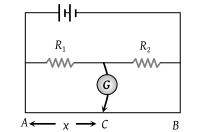(1) x

(2) x/4

(3) 4x

(4) 2x

Concept Questions :-

Meter bridge and potentiometer
High Yielding Test Series + Question Bank - NEET 2020

Difficulty Level:

Seven resistances are connected as shown in the figure. The equivalent resistance between A and B is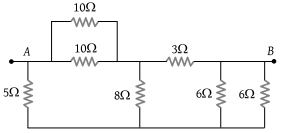(1) 3 Ω

(2) 4 Ω

(3) 4.5 Ω

(4) 5 Ω

Concept Questions :-

Combination of resistors
High Yielding Test Series + Question Bank - NEET 2020

Difficulty Level:

A battery of internal resistance 4Ω is connected to the network of resistances as shown. In order to give the maximum power to the network, the value of R (in Ω) should be :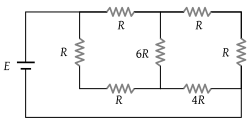(1) 4/9

(2) 8/9

(3) 2

(4) 18

Concept Questions :-

Combination of resistors
High Yielding Test Series + Question Bank - NEET 2020

Difficulty Level:

In the circuit shown here, the readings of the ammeter and voltmeter are [Kerala PMT 2002]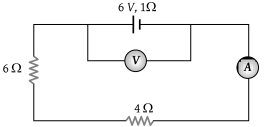(1) 6 A, 60 V

(2) 0.6 A, 6 V

(3) 6/11 A, 60/11 V

(4) 11/6 A, 11/60 V

Concept Questions :-

Kirchoff's voltage law
High Yielding Test Series + Question Bank - NEET 2020

Difficulty Level:

Length of a hollow tube is 5m, it’s outer diameter is 10 cm and thickness of it’s wall is 5 mm. If the resistivity of the material of the tube is 1.7 × 10–8 Ω×m then the resistance of the tube will be :

(1) 5.6 × 10–5 Ω

(2) 2 × 10–5 Ω

(3) 4 × 10–5 Ω

(4) None of these

Concept Questions :-

Derivation of Ohm's law
High Yielding Test Series + Question Bank - NEET 2020

Difficulty Level:

A wire of resistor R is bent into a circular ring of radius r. Equivalent resistance between two points X and Y on its circumference, when angle XOY is α, can be given by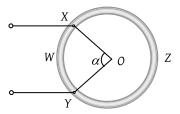(1) $\frac{R\alpha }{4{\pi }^{2}}\left(2\pi -\alpha \right)$

(2) $\frac{R}{2\pi }\left(2\pi -\alpha \right)$

(3) R (2πα)

(4) $\frac{4\pi }{R\alpha }\left(2\pi -\alpha \right)$

Concept Questions :-

Combination of resistors
High Yielding Test Series + Question Bank - NEET 2020

Difficulty Level:

The potential difference across the terminals of the battery shown in the figure is (r = internal resistance of battery) :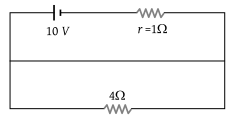(1) 8 V

(2) 10 V

(3) 6 V

(4) Zero

Concept Questions :-

Kirchoff's voltage law
High Yielding Test Series + Question Bank - NEET 2020

Difficulty Level:

As the switch S is closed in the circuit shown in the figure, the current passed through it is :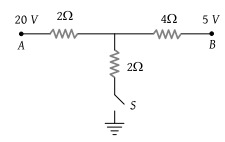(1) 4.5 A

(2) 6.0 A

(3) 3.0 A

(4) Zero

Concept Questions :-

Kirchoff's voltage law
High Yielding Test Series + Question Bank - NEET 2020

Difficulty Level:

In the following circuit, a 10 m long potentiometer wire with resistance 1.2 ohm/m, a resistance R1 and an accumulator of emf 2 V are connected in series. When the emf of the thermocouple is 2.4 mV then the deflection in the galvanometer is zero. The current supplied by the accumulator will be :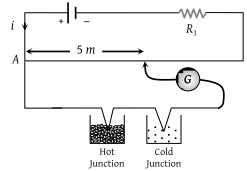(1) 4 × 10–4 A

(2) 8 × 10–4 A

(3) 4 × 10–3 A

(4) 8 × 10–3 A

Concept Questions :-

Meter bridge and potentiometer
High Yielding Test Series + Question Bank - NEET 2020

Difficulty Level:

In the following circuit, bulb rated as 1.5 V, 0.45 W. If bulbs glows with full intensity then what will be the equivalent resistance between X and Y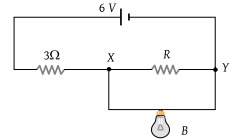(1) 0.45 Ω

(2) 1 Ω

(3) 3 Ω

(4) 5 Ω